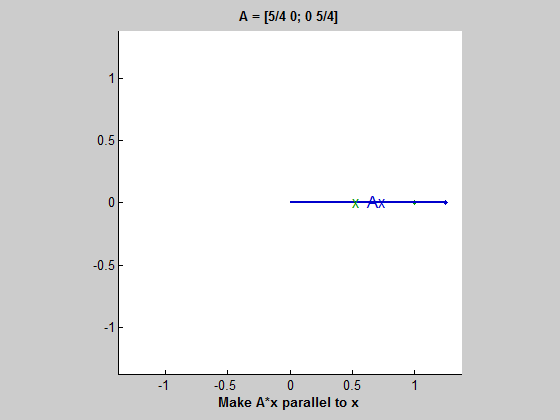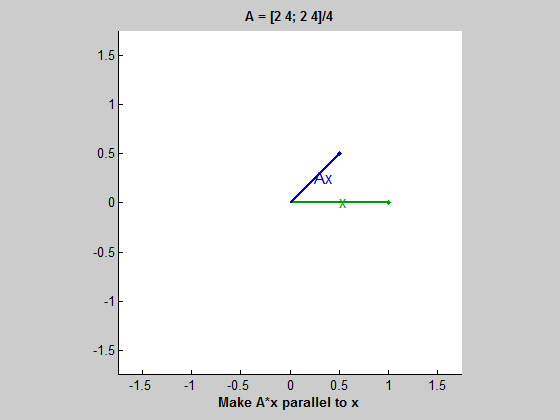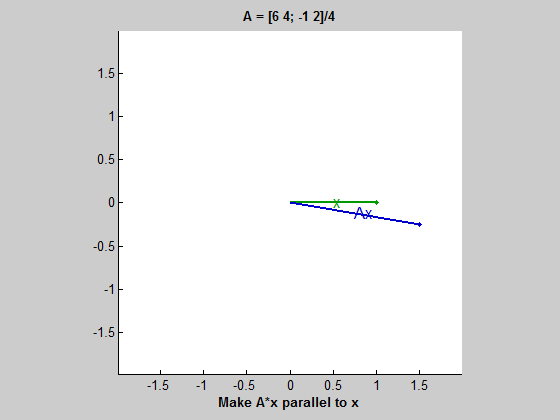# eigshow, week 2

Three more examples with eigshow, all of them degenerate in some way or another.

### Contents

#### A scalar matrix

The first example is a scalar multiple of the identity matrix. It is not available from the pull-down menu in the eigshow title, but eigshow can be called with a 2-by-2 matrix argument.

format rat, format compact
A = [5 0; 0 5]/4

A =
5/4            0
0              5/4The blue ellipse is a circle. Ax is always equal to (5/4)*x and so A*x is always parallel to x. Any vector is an eigenvector. 5/4 is a double eigenvalue. eigshow chooses two unit vectors as the eigenvectors but it could have chosen any two vectors.

#### A singular matrix

A = [2 4; 2 4]/4

A =
1/2            1
1/2            1The blue ellipse has collapsed to a line. It may be a hard to see when A*x is parallel to x, but it is when x itself is in the line, or when A*x is the zero vector. The corresponding eigenvalues are 3/2 (the half-length of the line) and zero. [Note added Aug. 5, 2013: This is not correct. See my comment posted today.]

#### A defective matrix

A = [6 4; -1 2]/4

A =
3/2            1
-1/4            1/2Matrices with this type of behavior are rare, but important. The blue ellipse is not degenerate, but as you move around the unit circle Ax lines up parallel for only one x and its negative. This is a matrix of order two with only one eigenvector. It has a double eigenvalue equal to 1 and a nondiagonal Jordan Canonical Form. Such matrices are known as defective and play an important role in the theory of ordinary differential equations and dynamical systems.

Published with MATLAB® R2013b

|